Courses

# Chemistry Test - 4

## 45 Questions MCQ Test | Chemistry Test - 4

Description
This mock test of Chemistry Test - 4 for helps you for every entrance exam. This contains 45 Multiple Choice Questions for Chemistry Test - 4 (mcq) to study with solutions a complete question bank. The solved questions answers in this Chemistry Test - 4 quiz give you a good mix of easy questions and tough questions. students definitely take this Chemistry Test - 4 exercise for a better result in the exam. You can find other Chemistry Test - 4 extra questions, long questions & short questions for on EduRev as well by searching above.
QUESTION: 1

Solution:
QUESTION: 2

Solution:
QUESTION: 3

### A → B   kA = 1015 e-2000/T C → D   kc = 1014 e-1000/T Temperature T/K at which (kA = kc) is

Solution:
QUESTION: 4

The vapour pressure of mixture of 1 mol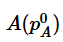= 150Torr) and 2 mol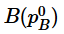= 240Torr) is 200 mm. In this case

Solution:
QUESTION: 5

In a first order reaction, A → P , the ratio of a/(a–x) was found to be 8 after 60 minutes. If the concentration is 0.1 M, then the rate of reaction in moles of A reacted per minute is

Solution:
QUESTION: 6

On adding AgNO3 solution into KI solution, a negatively charged colloidal sol is obtained when they are in

Solution:
QUESTION: 7

Most effective of electrolyte causing coagulation of a negatively charged As2S3 sol is

Solution:
QUESTION: 8

When 0.1 mol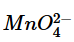is oxidised the quantity of electricity required to completely oxidiseto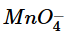is

Solution:
QUESTION: 9

Match the following correctly
Catalyst                        Industrial product
A. V2O5                        I. High density polythene
B. Ziegler-Natta           II. Poly acrylonitrile
C. Peroxide                 III. NH3
D. Finely divided Fe    IV. H2SO4

Solution:
QUESTION: 10

The rate constant for the reaction, N2O5(g) → 2NO2 +1/2 O2(g) is 2.3 x 10-2 sec-1. Which equation given below describes the change of [N2O5] with time [N2O5]0 and [N2O5]t correspond to concentration of N2O5 initially and time ‘t’ ?

Solution:
QUESTION: 11

A cell ; Cu/Cu2+ (1M) || Zn2+ (1M)/Zn should have cell voltage

Solution:
QUESTION: 12

Number of atoms per unit cell for body centred tetragonal system is

Solution:
QUESTION: 13

Maximum freezing point will be for 1 molal solution of, assuming equal ionisation in each case

Solution:
QUESTION: 14

Select incorrect statement

Solution:
QUESTION: 15

Which of the following arrangements shows schematic alignment of magnetic moments of antiferromagnetic substances ?

Solution:
QUESTION: 16

A button cell used in watches functions as following
Zn(s) + Ag2O(s) + H2O(l) → 2Ag(s) + Zn2+(aq) + 2OH- (aq)

If half cell potentials are
Zn2+ (aq) + 2e- → Zn(s); E0 = -0.76V
Ag2O(s) + H2O(l) + 2e- → 2Ag(s) + 2OH- (aq), E0 = 0.34V

Solution:
QUESTION: 17

Relation between atomic radius (r) and edge length (a) of a body centred cubic unit cell is

Solution:
QUESTION: 18

The emf of the cell Zn/Zn2+ (1M)|| X2+ (1M)/X is found to be 1.1V . If E�red of Zn2+/Zn is – 0.76 V, then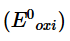of X is

Solution:
QUESTION: 19

If H2SO4 is added to water, then the solution

Solution:
QUESTION: 20

In a 0.2 molal aqueous solution of AB having degree of dissociation is 0.3, taking Kf for water as 1.85K molality-1, the freezing point of the solution will be nearest to

Solution:
QUESTION: 21

Which solution will show maximum elevation in b.pt.?

Solution:
QUESTION: 22

The freezing point depression constant for water is –1.860C m-1. If 5.00 g Na2SO4 is dissolved in 45.0 g H2O, the freezing point is changed by –3.820C. Calculate the van’t Hoff factor for Na2SO4

Solution:
QUESTION: 23

In Freundlich adsorption isotherm, the value of 1/n is

Solution:
QUESTION: 24

What will be the molecular weight of NaCl determined experimentally following elevation in the boiling point or depression in freezing point method ?

Solution:
QUESTION: 25

The rate law for the reaction;
RCl + NaOH(aq.) → ROH + NaCl is given by, Rate = K1 [RCl]. the rate of the reaction will be

Solution:
QUESTION: 26

During electrolysis of H2O, volume of H2 and O2 are released in the mole ratio

Solution:
QUESTION: 27

A first order reaction, which is 30% complete in 30 minutes, has a half-life period of

Solution:
QUESTION: 28

An endothermic reaction with high activation energy for the forward reaction is given by the diagram

Solution:
QUESTION: 29

In a gaseous phase reaction, A2 (g) → B(g) + (1/2) C(g) , the increase in pressure from 100 mm to 120 mm is noticed in 5 mins. The rate of disappearance of A2 in mm min-1 is

Solution:
QUESTION: 30

The rate constant of a reaction is 3 x 10-6 per sec. If the initial concentration is 0.10 M, the initial rate of reaction is

Solution:
QUESTION: 31

Which among the following is incorrect about Schottky defects ?

Solution:
QUESTION: 32

In which of the following reactions heterogeneous catalysis is involved ?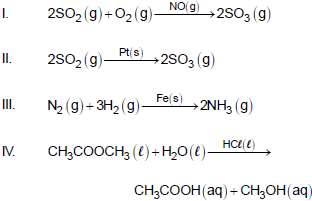Solution:
QUESTION: 33

The rate of disappearance of SO2 in the reaction; 2SO2 + O2 → 2SO3 ; is 1.28 x 10-3 g litre-1 sec-1.Then the rate of formation of SO3 in g litre-1 sec-1 is

Solution:
QUESTION: 34

Which of the following reactions is used to make a fuel cell ?

Solution:
QUESTION: 35

Bleeding is stopped by the application of ferric chloride. This is because

Solution:
QUESTION: 36

Which kind of defects are introduced by doping ?

Solution:
QUESTION: 37

The e.m.f., of a Daniel cell at 298 K is E1.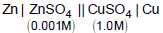When the concentration of ZnSO4 is 1.0 M and that of CuSO4 is 0.01 M, the e.m.f., changed to E2. What is the relationship between E1 and E2 ?

Solution:
QUESTION: 38

For a first order reaction A → B the reaction rate at reactant concentration of 0.01 M is found to be 2.0 x 10-5 M sec-1. The half life period of the reaction is

Solution:
QUESTION: 39

When an electric current of 0.5 ampere is passed through acidulated water for two hours, then volume of hydrogen (H2) at NTP produced at cathode will be

Solution:
QUESTION: 40

Electrode potential for Mg electrode varies according to the equation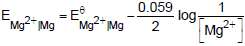.

The graph of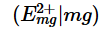vs log[Mg2+] is

Solution:
QUESTION: 41

Which of the following is correct statement for the spontaneous adsorption of a gas ?

Solution:
QUESTION: 42

Which of the following will behave as n-type semiconductor ?

Solution:
QUESTION: 43

Which one is not equal to zero for an ideal solution ?

Solution:
QUESTION: 44

In the closest packed structure of a metallic lattice, the number of nearest neighbours of a metallic atom is

Solution:
QUESTION: 45

Consider the first-order decomposition of A molecular in three vessels of equal volume.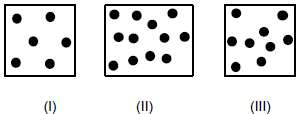Relative rates of decomposition in I, II and III are

Solution:

Track your progress, build streaks, highlight & save important lessons and more!

### Similar Content### Related tests